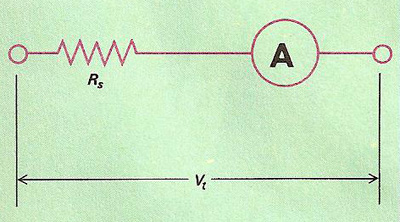A# voltmeterDC voltmeter circuit using a milliammeter (A) in series with a high resistance (Rs). If the resistance of the ammeter is known and the combined resistance of Rs and A is large compared with the resistance in the circuit under test, the ammeter reading allows a good estimate of the test voltage (Vt) to be made. For a particular ammeter-series resistance combination, the meter can be calibrated directly in volts.

A voltmeter is an instrument used to measure the voltage between two points in an electric circuit. Most consist of an ammeter connected in series with a high resistance and calibrated in volts. By Ohm's law the current flowing is proportional to the potential difference, though the instrument itself inevitably reduces the potential under test. Accurate dterminations of potential difference must employ a potentiometer.